# AP Chemistry : Buffers

## Example Questions

### Example Question #1 : Buffers

Which of the following can be used in a buffer solution?

HCl and HNO3

NaHCO3 and K2CO3

HNO2 and KNO2

NaOH and KOH

NaHCO3 and K2CO3

Explanation:

For a buffer solution, you need a weak acid and its conjugate base, or a weak base and its conjugate acid. HCO3 from the NaHCO3 and CO3– from K2CO3 are this pair.

### Example Question #1 : Help With Buffers

Which of the following would best buffer a solution from a pH of 4 to 6?

Uric acid (pKa = 3.9)

Carbonic acid (pKa = 6.3)

Hydrocyanic acid (pKa = 9.2)

Formic acid (pKa = 3.7)

Acetic acid (pKa = 4.7)

Acetic acid (pKa = 4.7)

Explanation:

A weak acid/base best buffers about 1 pH point above and below its pKa. The pKA closest to the middle of 4 and 6 (so want as close to 5) is acetic acid at 4.7.

### Example Question #3 : Buffers

Which of the following solutions has the greatest buffering capacity?

2M Formic Acid

4M Nitric Acid

3M Rubidium Hydroxide

1M Acetic Acid

2M Formic Acid

Explanation:

Nitric Acid is a strong acid and can't buffer. Rubidium Hydroxide is a strong base and thus can't buffer. Of the remaining, both are weak acids, but the one with a greater concentration has a greater buffering capacity.

### Example Question #1 : Buffers

To create a buffer solution, you can use a weak acid and                       .

another weak acid

its conjugate acid

its conjugate base

a strong base

its conjugate base

Explanation:

The definition of a buffer solution is that it contains a weak acid and its conjugate base, or a weak base and its conjugate acid. Since we are starting with a weak acid in this case, we need its conjugate base.

### Example Question #5 : Buffers

Which of the following will increase the pH of an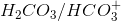buffer solution?

I. Removing carbonic acid

II only

I only

Both I and II

Neither of these options

Both I and II

Explanation:

To answer this question we need to look at the reaction below: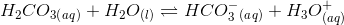An increase in the pH will result in a decrease in the concentration of hydrogen ions (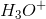). Using Le Chatelier’s principle we can find out which answer choices will decrease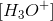.

Removing carbonic acid will decrease the concentration of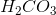. To maintain equilibrium, the reaction will shift to the left and make more reactants from products; therefore, there will be a decrease in theand an increase in pH.

Recall that salts like sodium bicarbonate, or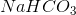, will dissociate in water and form ions. Sodium bicarbonate will form sodium (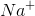) and bicarbonate (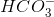) ions. This side reaction will result in an increase in the bicarbonate ion concentration. Le Chatelier’s principle will shift the equilibrium of the given reaction to the left and, therefore, decrease the. Adding sodium bicarbonate will increase the pH.

### Example Question #41 : Acid Base Chemistry

Which of the following combinations cannot be used to produce a buffer solution?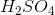and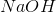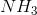and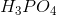and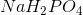andandExplanation:

Buffer solutions can be made via two methods. The first method involves adding equal amounts of a weak acid and a salt of its weak conjugate base (or vice versa). The second methods involves adding a weak acid and a half equivalent of a strong base (or vice versa).is a weak acid andis a salt of its weak conjugate base; therefore, this can form a buffer.is a weak base and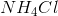is a salt of its weak conjugate acid; this can also form a buffer. Note that this is the converse of the first method (weak base with salt of weak acid), but it can still form a buffer solution.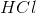is a strong acid andis a weak base; therefore, addingand a half equivalent ofwill create a buffer solution. This is the converse of the second method (adding a weak base to a half equivalent of strong acid).andare both strong reagents (acid and base, respectively); therefore, they cannot form a buffer solution.

### Example Question #6 : Buffers

Blood is a physiological buffer. The carbonic acid/bicarbonate system maintains blood’s pH at around 7.35. Carbon dioxide in blood undergoes a complex equilibrium reaction as follows: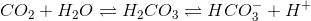Alterations to carbon dioxide levels can change the blood pH.

A patient has abnormally low levels of carbon dioxide in the blood. What can you conclude about this patient?

The patient has respiratory alkalosis (basic blood pH)

The body will compensate by decreasing the amount of bicarbonate

The body will compensate by increasing respiratory rate (hyperventilation)

The ratio of carbonic acid to bicarbonate will be decreased

The patient has respiratory alkalosis (basic blood pH)

Explanation:

The question states that the patient has low levels of carbon dioxide. If we look at the given reaction, we will notice that the reaction will compensate for this by shifting the reaction equilibrium to the left. This phenomenon is called Le Chatelier’s principle and occurs to maintain the equilibrium of the reaction; therefore, the reaction will create more carbon dioxide by utilizing bicarbonate and hydrogen ions in the blood. A decrease in hydrogen ion concentration in blood will increase the pH and cause alkalosis (basicity in the blood). Since carbon dioxide is the cause of alkalosis, this patient will experience respiratory alkalosis. If he experienced alkalosis due to a change in bicarbonate ion concentration, the patient will have metabolic alkalosis.

The ratio of carbonic acid to bicarbonate will stay the same because both will be used in equal amounts (1:1 ratio) to produce carbon dioxide. Increasing respiratory rate, or hyperventilation, will result in an increase in the amount of carbon dioxide expelled by the patient; this will decrease the carbon dioxide concentration in the blood and will worsen the respiratory alkalosis. Recall that we are utilizing the bicarbonate ion (in conjunction with hydrogen ions) to create carbonic acid. The carbonic acid will be further broken down to replenish the carbon dioxide. A decrease in the bicarbonate concentration will slow down this process.

### Example Question #42 : Acid Base Chemistry

A researcher is trying to make a buffer solution from a weak acid and its weak conjugate base. The pKa of the acid is 5.9 and the desired pH of the buffer solution is 3.5. Which of the following is the best way to make this buffer solution?

Add a 1:1 ratio of the weak acid to its weak conjugate base

Add a 1:1 ratio of the weak acid to a strong base

The researcher cannot use this weak acid to make the desired buffer solution

Add a 2:1 ratio of the weak acid to a strong base

The researcher cannot use this weak acid to make the desired buffer solution

Explanation:

One way to make a buffer is by adding equal amounts of a weak acid to its weak conjugate base. For example, you can add 1M acetic acid to 1M acetate to create a buffer solution (note that both acetic acid and its conjugate base (acetate) are weak). However, when using this method you have to remember that the desired pH of the buffer solution has to equal the pKa of the weak acid. The question states that the pKa of the acid is 5.9 and the desired pH of the buffer is 3.5; therefore, it is not possible to make the buffer with the given acid. The researcher would have to find another acid that has a pKa near 3.5.

### Example Question #7 : Buffers

There are many solution systems which can only function as desired when the pH of that solution stays within a narrow range. Maintaining a stable pH in an unstable environment is most often achieved by the use of a buffer system, which is composed of a conjugate acid-base pair. One physiologically important buffer system is the bicarbonate buffer system that resists changes in blood pH.The acid dissociation constant of carbonic acid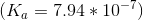The normal blood pH is tightly regulated between 7.35 and 7.45

When blood pH falls below 7.35 a person is said to have acidosis. Depending upon how far the pH drops, this condition could lead to nervous system impairment, coma, and death.

What is the ratio of bicarbonate ion concentration to carbonic acid concentration at which an individual will be at the threshold of experiencing acidosis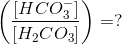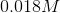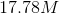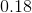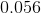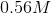Explanation:

###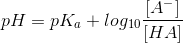To use this equation we need to convert the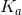to the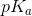, and can use the following definition to do so: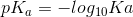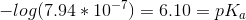Since an individual will begin experiencing acidosis when the blood falls below 7.35 we can use 7.35 as the pH in the Henderson-Hasselbalch equation. Adding in theof 6.10 calculated from thegives the equation below: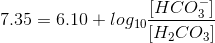###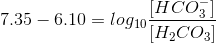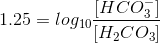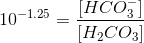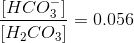The answer is unitless because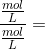all units cancel out.

### Example Question #1 : Buffers

A buffer using acetic acid (pKa=4.76) is titrated with NaOH. What is the pH at half the equivalence point?

2.38

4.76

12.36

7.00

9.52